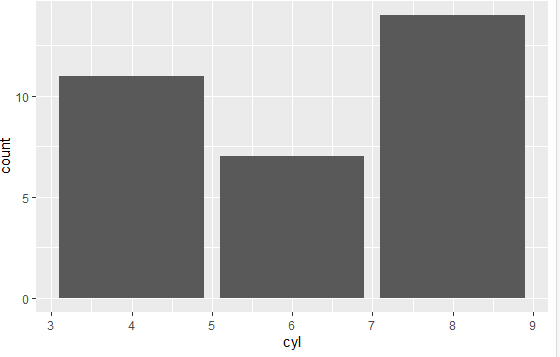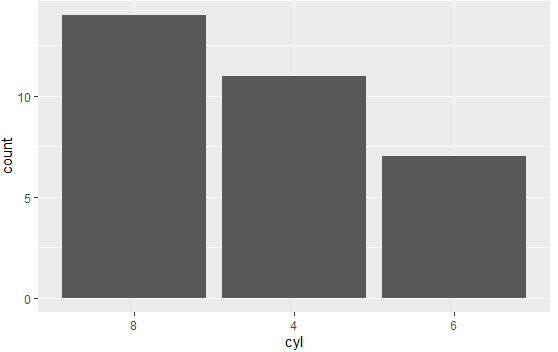2 views

I am making a dodged bar chart using ggplot with discrete x scale, the x axis are now arranged in alphabetical order, but I need to rearrange it so that it is ordered by the value of the y-axis (i.e., the tallest bar will be positioned on the left).

I tried order or sort, but result in sort the x-axis, but not the bars respectively.

What have I done wrong?

by

To change the order of the discrete x scale, you need to factor the variable and set the levels of the variable that you are plotting.

For example:

In mtcars dataset:

library(ggplot2)

# Automatic levels

data(mtcars)

ggplot(mtcars, aes(cyl)) + geom_bar()

Output:To factor and set the levels:

mtcars\$cyl <- factor(mtcars\$cyl, levels = names(sort(table(mtcars\$cyl), decreasing=TRUE)))

ggplot(mtcars, aes(cyl)) + geom_bar()

Output: